# Spiral Features

## Tree of Life

There is a collection of esoteric sciences that can collectively be referred to as the Metatronic sciences.  These represent a digression from earlier Krystic sciences.

In particular the Metatronic Tree of Life derives from the earlier and more complete 12 Tree Kathara Grid.  This is illustrated in the diagram over.  In comparison with the Kathara Grid we see that centers 8 and 12 are missing in the Metatronic Tree of Life.  These are key grid connection points.The Krystal spiral has direct relationship with the Kathara Grid.  We have seen in the development of the Metatronic / Fibonacci Spiral above how this spiral can have impacted these key 8 and 12 centers in the original Kathara Grid leading to the reduced 10 center Metatronic Tree of Life.

The dimension 5 center (original Kathara 5) forms a new false center in the Metatronic Tree of Life grid.  This differs from the Central Seed Atom at the center of the Kathara Grid.

The Metatronic grid is associated with a ‘floral’ pattern.  There is a significance to this, which will be discussed below.  This causes the grid to be narrower than the original Kathara Grid.  The width is reduced to 87% of the original width.

Center 1 is pulled down to fit the floral pattern.  The height to width ratio of the grid is 4 / √3   = 2.3 instead of 2.  This distorts the balance and proportion of the grid.

The vertical symmetry of the grid is broken.  In other words the upper portion differs significantly from the lower portion.  The original Kathara Grid is completely symmetrical about its center.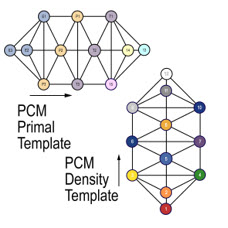The Kathara Grid is a fundamental and very practical structure in creation.  Effectively it’s a map of dimensional structure.  It carries the encryption or core design for manifestation within 12 dimensions in creation.  Its structure and proportions are key to natural creation.  The Kathara Grids from different levels in creation are capable of aligning to allow open flow between these levels.  In particular the Kathara Grids are capable of aligning end to end at 90° angles to form the 4 quadrants in a Veca universe, as illustrated in the diagram.  This is possible because of the symmetry between the top and bottom of the Kathara Grids and the angular alignments of the centers.

Furthermore the Kathara Grids at 90° angles can be overlaid accurately one on top of the other because the central portion is a true square.  This allows for normal interaction between the Particum (PCM) matter side of a universe and its parallel PartikA (PKA) side.  This is illustrated in the overlaid grids in the diagram above.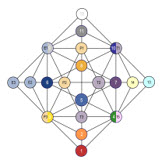What happens if we try to align the Metatronic grids on a similar basis?  This is illustrated in the diagram over.  Here we see that the Metatronic grids are incapable of aligning end to end on 90° alignments due to the lack of symmetry between the top and bottom, missing centers and angular distortions.  This means that different levels of creation based on such structures are incapable of opening to each other.  The do not allow free passage between such levels.

Also if we try to overlay Metatronic grids at 90° angles directly on each other we find that there is no consistent alignment between the centers of the two grids.  This is illustrated in the diagram over.  Here again the lack of symmetry and the difference in center spacing between horizontal and vertical directions do not support alignments.  The distortion of the grid proportions has a serious impact here.  This means that the matter (PCM) and parallel (PKA) grids in a local universe under the influence of such grids cannot interact naturally.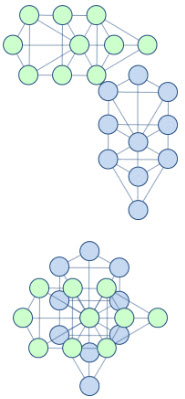Such conditions lead to a closed and isolated local universe.  This is neither how it started out originally nor how it was intended to be.

## Lotus Breathing

The Kathara Grid is a living structure.  It holds a direct connection to Source through its Central Seed Atom and is continually interacting with Source through this point.  There is a natural breathing pattern in the Kathara Grid that draws streams of life force current from Source through the center.  It distributes this current through the grid and returns the current back to Source through the center.  This is referred to as the Lotus Breathing pattern.  It is illustrated in the diagram.

The blue petals represent the streams of current and are referred to as the Lotus petals.  As the breathing pattern progresses the outer petals at 45° angles on either side close over the central vertical petals on an inhale.  On an exhale they open out again to a maximum extension of 45° angles on either side of the central vertical column of the grid.  Thus the 9 & 10 center petals close over the 11 center petal on an inhale and open back out again for an exhale.  The lower petals move similarly in synchrony with the upper ones.  This phasing of the petals draws current from the center on an exhale and brings it back in on an inhale.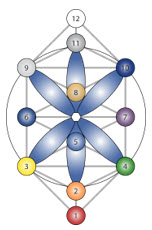The outer petals only extend to a maximum angle of 45° from the central vertical column.  This is important.  It allows space at the 6 and 7 center positions for a similar set of petals to phase for the parallel grid on a 90° alignment.  These two sets of parallel Lotus petals PCM & PKA phase rhythmically with one set open while the other is closed and vice versa.

In the Metatronic grids there is a sextant distortion where the lotus petals are pulled beyond their 45° natural extension and are locked open in a forced 60° extension.  This traps the life force energy within the grid.  However, it also stops the regular breathing and prevents the flow of fresh current from the center and its subsequent return to Source.  This set of petals in 60° extension gives us the floral motif underlying the Metatronic grid.Each such floral unit represents a quantity of trapped life force.  These are overlaid to form a grid pattern as shown.  This represents a static life force field rather than a natural breathing system with connection to Source.  Each of the Metatronic grid centers in turn lies on one of these floral units and is sustained by it.  The Metatronic Tree of Life centers are moved to overlay the floral pattern.  This leads to significant distortions in the grid with results as discussed above.

## Spiral Number Formation

The numbers associated with these spirals have some further interesting information to impart.

Geometrically, spirals start with a base size of 1.  The Krystal Spiral expands by √2 = 1.414… every 45° and by 2 every 90°.  The spirals are continuous curves and expand through every number from 1 upwards.  However we are not interested in all the numbers, only the ones at certain key points.  For example in driving from New York to Washington we pass through every point along the way, but are only interested in the distances at key points e.g. Philadelphia, Baltimore, etc.  For spirals generally the most important points are at 90° intervals.  These are the quadrant points.

For the Krystal Spiral the numbers for the key quadrant points are 1, 2, 4, 8, 16, 32… as indicated previously in the Spiral Numbers table.  The corresponding quadrant numbers for the Fibonacci spiral are 1, 1, 2, 3, 5, 8 …

The Fibonacci numbers can be developed by a process of addition.  Starting from the simplest numbers, 0 & 1, add progressive pairs to get the next number.  Every number is the sum of the previous two.  The diagram over illustrates the growth of this sequence in successive stages.

The ratio between each pair of numbers approaches the Golden Mean ratio e.g. 55 / 34 = 1.618…  The numbers from 1 outwards correspond with the Fibonacci Spiral.  The numbers beyond the first few terms are equivalent to a scaled down version of the Golden Mean Spiral, as mentioned previously.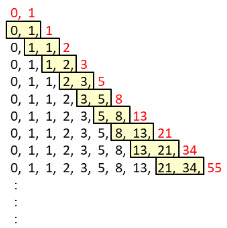As we can see, each number has connection to the previous two only.  There is progressively greater isolation from the source numbers and particularly from the zero point Source.  The corresponding numbers for the Golden Mean Spiral would display similar behaviour.

What would happen if, instead of adding the previous two numbers, we added the previous 3?  In this case we would get a sequence of numbers where the ratio between successive terms approaches a value higher than 1.618… but less than 2.  As we add progressively more terms this ratio gets ever closer to 2.

Now what would happen if instead of adding just the previous two we added all the previous numbers?  The result is illustrated in the diagram below.  Here the numbers sum to 1, 2, 4, 8, etc. at successive stages of development.  We see that we get the Krystal Spiral numbers.  Here we have the zero point at the beginning and an additional 1.  Effectively each Krystal Spiral number is the sum of all the prior numbers plus unity.

The ratio between successive numbers is the Krystal spiral ratio of 2.  This illustrates that the Krystal Spiral can be generated by a process of addition as well as by multiplication.

Let’s look more closely at the unity (1) in the Krystal number sequence.  All natural spirals are capable of contracting inward from 1 towards the center in addition to expanding outwards.  Each can spiral inwards around its center getting ever closer to the center, i.e. generating an inner spiral.  This is illustrated by the dashed curve in the diagram over.  The center that the spiral rotates around and expands from corresponds to the number zero.  The zero point is the center and holds direct inner connection to Source.

The processes of outward expansion and inward contraction are complementary.  For example, we can look at creation as a process of dividing the inherent unity of Source into progressively smaller units.  As these units get smaller they are also getting more numerous.  We have the two processes working side by side here.  Multiplication of diversity and division of unity are complementary processes.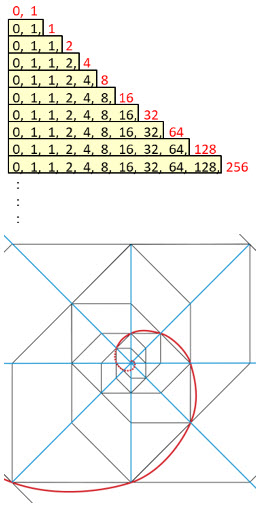If we continue the Krystal spiral inwards we progressively divide by 2 instead of multiplying by it.  This will generate a sequence of reducing numbers as follows – ½, ¼, 1/8, 1/16, etc.

However all these fractional numbers sum to unity.  Unity is the sum of all the numbers for the inner spiral to the zero point at the center, i.e.

1  =  1/2 + 1/4 + 1/8 + 1/16 + . . .

Now we can replace the extra 1 at the beginning of the Krystal sequences above with this fractional expansion to get for example: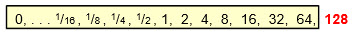So every Krystal number is the sum of all the previous numbers including the inner spiral, all the way towards the center.  Effectively every point integrates and preserves the content of all the previous points.

More generally, every point on the Krystal Spiral is the sum of all the inner quadrant points from there to the center.  For example, we can take the expansion 1  =  1/2 + 1/4 + 1/8 + 1/16 + . . .   and multiply it by any number.  Take 56 for example:

56  =  56/2 + 56/4 + 56/8 + 56/16 + . . .

Now with division by two 56/2 lies one quadrant (90°) back from 56 on the Krystal spiral.  56/4 is a quadrant earlier again and likewise with all the other elements in the expansion.  This means that every number or point on the Krystal spiral is the sum of all the prior quadrant points all the way back to the center.

We can visualise this property in graphical terms as follows.  Take any point on a Krystal Spiral (A) and draw an axis through it to the center and out the other side as illustrated in the diagram over.  Draw another line through the center at 90° to this.  This gives us 4 quadrant axes spaced 90° apart, as illustrated by the dark blue lines.  The chosen point (A) will be the sum of all the inner spiral intersection points with these axes (1, 2, 3, 4, …) into the center.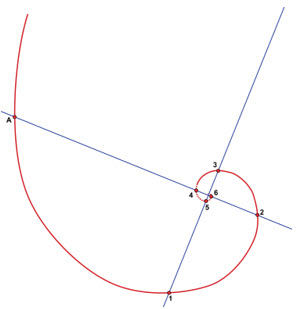Every point on the Krystal spiral thereby holds direct connection to Source.  Every point also integrates and preserves the content of the previous points.

These powerful features are unique to the Krystal Spiral.

## Spiral Features

Spirals combine two of the most fundamental processes in creation.  They combine rotation with expansion and contraction.  This is the context in which we can speak of spirals of creation.

The simplest form of rotation is circular motion.  This is illustrated in the diagram below.  In practical terms this could be a weight rotating on the end of a string. over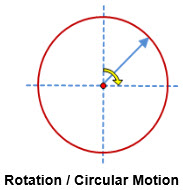Circular motion generates oscillations or repetitive wave patterns.  This is illustrated in the second diagram below.  If we take the arm ‘0 – a’ and look at the shadow it casts on the vertical axis ‘-1, 0, 1’ as it rotates, we see that the shadow of point ‘a’ will move up and down the axis.  This point ‘y’ will oscillate along the axis.  If we expand out this motion, e.g. by tracing it out over time, we see that it will trace a regular wave pattern, as illustrated to the right of the diagram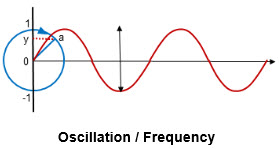Circular motion generates such wave patterns or oscillations.  This is demonstrated in practical terms by the way in which the rotation of an electrical generator produces waves of oscillating electrical current.  Any cyclic activity such as the cycle of the seasons, day night etc. derives from circular motion.  Oscillatory activity is all around us e.g. the sound tones of our speech, music, radio waves, waves on the ocean, the pulse / beating of our heart etc.

All oscillation or indeed cyclic behaviour is associated with circular motion at some level.  Oscillation and circular motion are synonymous.

The frequency of oscillation is associated with the speed of the circular motion.  One complete cycle or wave corresponds to a rotation of the circle.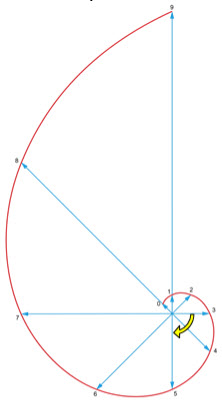If, instead of keeping the length of the arm or radius constant as it rotates, we allow it to expand we get a spiral.  This is illustrated in the spiral diagram, where the arm expands or grows out from the center to trace progressive stages of the Krystal spiral.  The rate of expansion is keyed to the rotation i.e. we have a doubling for every 90° of rotation in this case.  Moving in along the spiral towards the center we would have a similar contraction.

The spiral therefore combines the two fundamental processes of growth and oscillation.

## Growth & Decay

Growth processes share a common behavior.  This applies whether it is growth in nature  e.g. of trees,  population growth, growth of money, economic growth etc.  Growth arises through multiplication.

If we take money, for example, and for the sake of simplicity assume we have an excellent investment that doubles in value over a year.  We start with say \$1,000.  After year 1 this will have doubled to \$2,000.  After a second each of those thousands will have doubled so that we have \$4,000.  After a third year we have \$8,000 and so on.  The growth arises by repetitive multiplication by 2.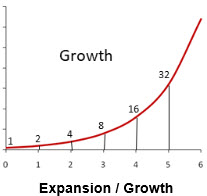The same principle would apply even if the annual return was say 5%.  In this case we would multiply be 1.05 for the first year, repeat this for the second year, etc.  So at the end of 4 years for example we would have 1.05 x 1.05 x 1.05 x 1.05 times the original amount.  We have repetitive multiplication over the 4 years.

Such repetitive multiplication is called exponentiation.  Just as repetitive addition gives us multiplication, repetitive multiplication produces exponentiation.

Such growth is referred to as exponential growth.  It is the same in principle as the expansion we get with the spirals.  We have repetitive multiplication in both cases.  The rate of growth may vary but the principle is the same.  Spirals that expand by repetitive multiplication are referred to as exponential spirals.  The Krystal and Golden Mean Spirals are exponential spirals directly and the Fibonacci Spiral progressively becomes one, after the initial stages.

A feature of exponential growth is that as the growth increases it gets faster.  This is illustrated in the diagram above.  For example the more money one has the faster it grows!  The present world population took a long time to reach the first billion, whereas the last billion was added in a few decades.  There is a ‘natural’ growth factor (e = 2.718.) where the rate of growth equals the state of growth.

The reverse process to growth is one of decay or contraction.  We have examples in the cooling of a cup of coffee, decay of the sound from a guitar string, radioactive decay etc.  Again the principle is the same.  We have repetitive division.  Also as decay progresses it moves more slowly, as illustrated in the diagram.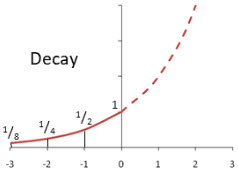The same (exponential) curves apply to both processes.  One process is going in the direction of positive numbers while the other is going in the negative direction.

Such growth and decay is encompassed in the spirals of creation.

## Spiral Relationship with Origin

We have seen above that regular spirals in addition to expanding outwards can contract inwards, spiralling ever closer to the origin at zero.  The spiral always remains positive and only approaches zero after an infinite number of steps on the inner / negative or decay direction, as illustrated in the Decay diagram above.

The Fibonacci Spiral’s approach to zero and inner progression is different.  The numbers for the inner stages of spiral progression are listed in the table above.  Here we see that the Krystal and Golden Mean Spiral numbers remain positive but get progressively smaller as we move further through negative stages in the inner direction.  They approach zero after an infinite number of stags.  This arises because these are both regular spirals.  The Fibonacci Spiral initially passes through zero but then starts oscillating between positive and negative values.  These oscillating values increase in the inner direction.  They ultimately diverge towards infinity instead of approaching zero.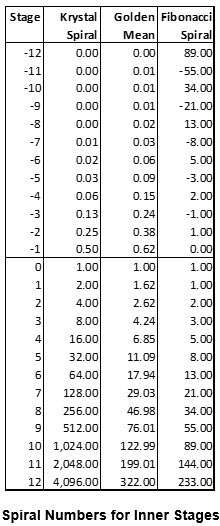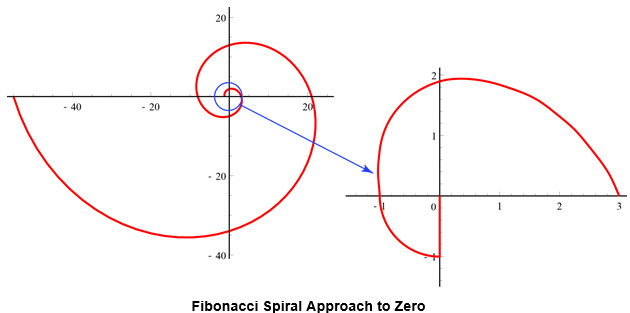The behaviour of the Fibonacci Spiral as it approaches zero is illustrated in the diagram above.  We can trace the inner contraction of the spiral by going in a counter clockwise direction along it.  The stages for the bigger numbers are illustrated in the left portion of the diagram.  As the spiral approaches zero its behaviour is magnified in the right portion.  Here we see the spiral passing through 3, 2 &1.  It then hits 1 a second time and drops down to zero.

Once we pass through the zero point towards the inner stages the spiral starts to oscillate between negative and positive values.  This behaviour cannot be illustrated readily on a spiral diagram like that above.  The negative radii are difficult to interpret and represent.  One possible interpretation is to consider the inner spiral as another spiral expanding in the opposite direction of rotation.  Instead of approaching zero in the inner direction it oscillates back out to infinity.

The behaviour of the spiral can be represented in simpler graphs, where we just show the magnitude of the spiral at each stage and ignore the rotation aspect.  This is illustrated in the diagrams below.  The Fibonacci Spiral is shown in red and the corresponding Golden Mean spiral in green for comparison.

Here we can see the increasing oscillation of the Fibonacci Spiral as it progresses to the left through negative stages in the inner direction.  This contrasts with the smooth progression of a regular spiral.

A regular spiral also has a constant rate of expansion in the outer direction and similar rate of contraction in the inner direction.  For the Krystal Spiral this rate of expansion from stage to stage is a factor of 2.  For the Golden Mean Spiral it is a factor of Φ  = 1.618…The Fibonacci Spiral approaches a constant rate of expansion similar to that for the Golden Mean Spiral, as it matures towards a stable spiral in the stages of expansion beyond say 6.  This is illustrated in the diagram above over.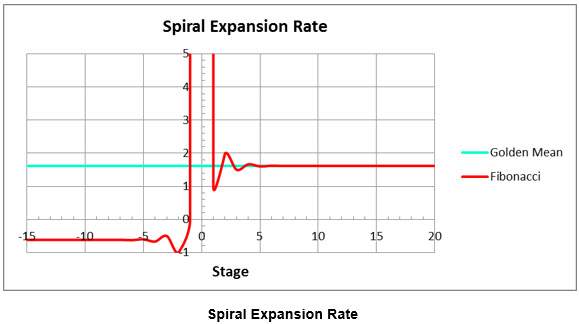The rate of expansion for the Fibonacci Spiral at each stage is shown in red.  The similar rate for the Golden Mean Spiral is shown in green for comparison.

Here we see that the rate of expansion becomes unstable in the vicinity of stage zero. It starts oscillating.  It goes to infinity at stage zero corresponding to a sudden expansion in magnitude from zero to 1.  In the inner / negative direction the rate of expansion goes negative and oscillates for a bit before settling down to a value of -1/Φ  =  -0.618… It’s interesting that this ratio has a complementary relationship to the normal value of Φ  = 1.618…  They are both roots of the formula that defines Φ, i.e. Φ2 = Φ + 1.

This illustrates that from stages 6 or so onwards the Fibonacci Spiral approaches the behaviour of a regular spiral.  However, before this stage its behaviour is erratic.  In the vicinity of zero it doesn’t behave like a spiral.  No regular logarithmic spiral that expands (or contracts) by multiplication can pass through zero value.  A zero multiplier anywhere in the chain of multiplication pulls all values down to zero.  Such a spiral cannot suddenly jump from zero to one in physical terms.  In the inner direction the oscillation isn’t normal behaviour for a regular spiral.

The Fibonacci Spiral therefore isn’t a proper spiral in its early stages.  It needs to be generated or kick started by some other process and as it stabilises it then approximates a scaled down version of the Golden Mean Spiral.

## Creative Play of Numbers

Numbers play a very significant role in the unfolding of creation.  They carry much information, structure and consciousness.  If we can give them voice and hear what they have to say they have a very interesting story to relate.

Ordinary numbers can be represented geometrically as spread out along a line like a ruler.  The positive numbers are shown progressing to the right.

Negative numbers are also important.  They become very important if our bank account is in debt for example!  We get the negative numbers by multiplying the positive ones by -1.  Geometrically this is equivalent to swinging the number line ‘0 – X’ above through 180°.  This gives the collection of what are referred to as real numbers.

Are there any other numbers of interest between these positive and negative numbers?  In other words if we were to swing the number line through an angle other than 180° would we get anything meaningful?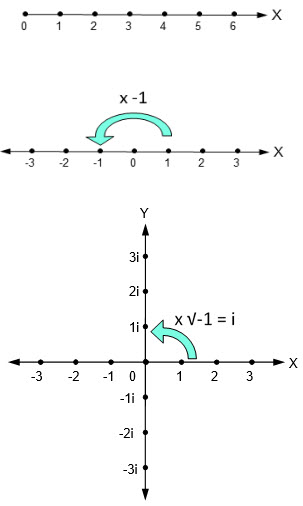The answer to this is a definite yes.  It turns out there is a very important and useful set half way between the two at 90°.  This corresponds to the ‘Y’ direction in the diagram above.

We get these numbers by doing a half step multiplication by  -1.  The half step in multiplication by -1 is √-1.

Therefore to rotate the number line above through the 90° of interest we multiply all the numbers by √-1.  This is a very important number and is given its own special shorthand symbol, i = √-1.  Therefore we show all the numbers on the 90° direction or ‘Y’ axis above as multiplied by i, which is understood as √-1.

Are these new numbers useful, significant or interesting?  It turns out that they are of huge importance and play a major and exciting role in the unfolding of creation.  They bring a level of sophistication that opens a vast new playground for creation to explore.

Now √-1 presents us with an enigma.  When we square any real number, i.e. any number on the real number line above, we always get a positive number.  Multiplying a positive number by itself gives a positive number.  Likewise multiplying any negative number by itself also yields a positive result, e.g. -1 x -1 = 1.  There is no real number that will square up to a negative number, in particular none that will square to -1.  So √-1 and all the associated ‘Y’ axis numbers don’t relate to any real numbers!  Although very useful, nobody knows where they come from.  They are described as imaginary.  Hence the ‘i’ symbol used to denote them.  Nonetheless their role and impact are both real and significant.  The collection of real and imaginary numbers are referred to as complex numbers.  Where do they come from?

If we recall the Partiki Phasing process we can see where these so called imaginary numbers come from.  This process is described in the Kathara 1 documentation [Reference 1].  We can summarize it briefly as follows.

The essence of creation is Source bringing focus of attention to a particular idea and in so doing creating structure around it.  The early manifestation of such structure arises as a non-polarized or omni-polar expression that carries the original thought or intention as an encryption.  This is represented as the Partiki (PKI) expression in the diagram over.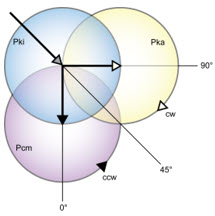The creative process advances within the Partiki unit to create a replica expression.  This replica in turn polarizes to manifest two opposite expressions of the original theme or idea.  These emerge from the Partiki unit and spread apart until they are at 90° to each other and 45° to the Partiki source.  These units carry opposed polarities.  They can be described as electrical PartikA (PKA) and magnetic Particum (PCM), or as matter and anti-matter, parallel worlds etc.  These polarities interact to weave the fabric of creation.

Having reached maximum expression as shown above, the polarized PKA and PCM units draw together and resolve their polarities.  They merge and integrate back into the original PKI unit.  This in turn then dissolves back to Source to release the structure and complete the cycle.  The process can then commence again for a new cycle of expression.  Such repeated Partiki phasing over many such units acting in harmony and over many cycles manifests creation.

We now see that the real and imaginary numbers derive from a similar process of polarization.  They are polarized expressions of each other and of a parent number sequence.  The 90° alignment arises from this polarization.  They interact to manifest additional structure in creation.  We can regard the real numbers as a PCM sequence and the imaginary ones as the corresponding PKA numbers.
The Partiki phasing process comes right through in the numbers!  These numbers embody the polarized structures in creation and illustrate their dynamic in a very powerful and beautiful way.  This concept of polarized numbers and structures is of fundamental importance and is key to understanding the power of numbers and the role they play in creation.

Now we have seen already that exponentiating or repeatedly multiplying a certain base number or growth rate a real number of times gives us growth in the positive direction and decay in the negative direction.  What happens when we exponentiate similarly with the imaginary ‘i’ numbers?  Here’s where something quite unexpected and totally magical happens.

Instead of growth and decay we get circular motion.  In other words when we exponentiate with the imaginary numbers the resulting numbers move around a unit circle, shown in blue in the diagram above.  This is fundamentally important as such circular motion underlies all vibratory/oscillatory activity.  Effectively we get oscillation and frequency.  We need these imaginary numbers to describe all oscillatory activity.  This is why they are so useful in practice.

Thus the same underlying process gives us both growth/decay and oscillation/ frequency.  We get one or the other depending on which set of numbers we use.  The real/PCM numbers give us growth while their imaginary/PKA counterparts generate oscillation.  This means that these two processes are polarized expressions of each other.  The spiral integrates them.  It is the PKI omni-polar host or parent.  This sequence illustrates how the spiral generates and regulates the fundamental processes of growth/decay and of oscillation in creation.  These are the spirals of creation.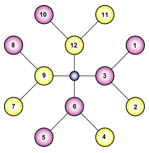## Number Formation

There is structure in the emergence of number.  This is illustrated very well in the Reuche formation, illustrated in the previous diagram above.  Here we have the omni-polar core at the center that holds direct connection to Source.  This produces two polarities represented by the 12 and 6.  It produces a further set of polarities at 90° to this as the 9 and 3.  These provide us with the four primal quadrants.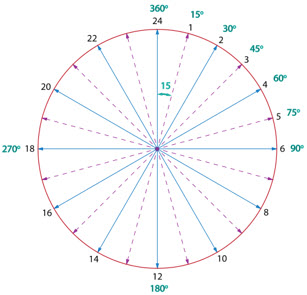Each of these in turn polarizes further to generate 8 additional numbers further out.  These represent the octants.  Collectively they provide 12 numbers, comprising four sets of three.  12 is a key number in Krystic structure.  We have 12 centers in a Kathara grid, 12 Allurean chambers etc.  The 12 numbers can also be arranged in clock formation, uniformly spaced around a circle.

Wherever we have a 12 structure we often have a second 12 in association with it.  For example we have two 12 Tree Kathara Grids aligned in relationship to form a Time Matrix.  We have 12 Allurean chambers with 12 Fire chambers aligned in between them.  These are distributed around a circle to give us a 24-hour clock.  It is interesting that the daily cycle is also divided into 24 hours.  Correspondingly we have 24 time zones distributed around the planet.

15 is also an important number that arises frequently in Krystic structure.  We have 15 dimensionalized dimensions in a time matrix, 15 chakras, 15 Hova/aura body levels etc.  Also where the original numbers are given in the Ma-ShA-Ya-Nic Aurora Code over, we have 15 specific numbers enumerated, in addition to the zero.

Now if take our 24-hour clock and sub-divide the sectors by 15 we get the 360° pattern in a circle or cycle as shown above.   It is interesting that this 360 division also approximates the number of days in a year.  Some pre-historic records e.g. the Dendera zodiac, indicate a 360 day year.  In that case the sun would move through one such degree in the sky each day.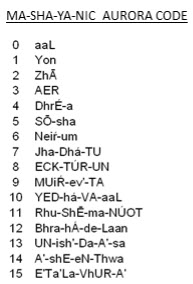## System Dynamics

We now have all the basic building blocks in place to explore system dynamics.

Take any system, a guitar string for example.  When we pluck the string we stimulate the ‘system’ by feeding energy into it.  This energy will gradually dissipate due to friction effects, resistance etc.  It usually ends up ultimately as waste heat.  The process of resistance opposes the stimulus and dissipates its energy.  We can show these two opposed processes at work on the horizontal line in the diagram below.

However there are additional effects at work in the system that provide for more interesting behaviour.  Any physical system will have some degree of inertia and also a certain elasticity or springiness.  These elements absorb the input energy from the stimulus and store it within the system.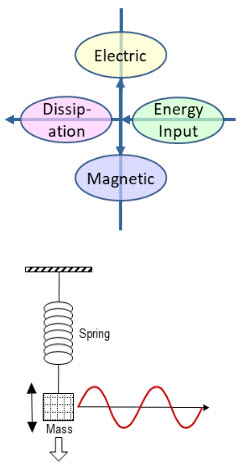For example the guitar string will have a certain weight and an elasticity determined by its tension.  The situation is a little clearer if we consider a weight suspended from a spring, as in the second diagram below.  If we stimulate the weight with an impulse it will bob up and down on the spring, setting up an oscillatory motion.

Some of the energy input to the system is used to stretch the spring and is stored in the spring.  Some is used to set the weight in motion and is stored in this motion (as kinetic energy).  Effectively the energy is stored within the system between the motion of the weight and the spring.

These energy storage effects arise at 90° to the original impulse.  Furthermore the two storage effects are opposed to each other.  When one is giving out energy the other is absorbing it and vice versa.  What we get then is an exchange of energy between the inertia of the weight and the elasticity of the spring – they cycle the stored energy between them.

This cycling sets up an oscillation.  The frequency of the oscillation depends on the balance between the inertia and elasticity in the system.  There is one particular frequency at which the energy storage capacities of the weight and the spring exactly balance each other.  This is known as the resonant or “natural” frequency of the system.  If we stimulate any system it will tend to oscillate at this natural frequency.  For example, if we pluck a guitar string it will oscillate at a particular frequency and generate a corresponding sound tone.  We can change this frequency by adjusting the tension (elasticity) of the string.  We can also change it by using different weights of string e.g. heavier strings.  There are various ways we can adjust the parameters to get a wide variety of frequencies and sound tones.

So, when we stimulate a system, effects operating at 90° to this set up an oscillation in the system.  In the absence of other influences this oscillation would continue indefinitely.  However, in our ”real” world we normally have some resistance or friction in the system, generally referred to as “damping”.  This draws energy out of the system and dissipates it.  It acts in opposition to the original stimulus as shown in the diagram above.  The oscillation is damped and dies out.

In our present world a lot of the energy leaving systems (to the left in the quadrant diagram above) is wasted.  A significant portion ends up as low-grade heat.  In a Krystic world this energy would cycle back as back-flow return to Source.  It would then re-emerge and come available again.  This is the ultimate in re-cycling and sustainability!

Any oscillation is cyclic in nature and can be described in terms of circular motion.  Different effects are dominant at different points or phases in the cycle.  The angles between these phases are important.  These angles are represented in the diagram above.  These effects are universal and arise in the dynamics of any system, mundane to cosmic.  No matter how complex the system, the fundamental behaviour is the same, whether it is a sound system, mechanical system e.g. the vibrations of an engine, electrical system e.g. power oscillations, electronic system, radio waves and so on.

In a more general sense, the quality of elasticity is electrical in nature, while that of inertia is magnetic.  So, more generally, oscillations are determined by a balance between the electrical and magnetic qualities of a system.  These two qualities are opposed to each other and exchange energy between them, to set up oscillations in a system.  The frequency of the oscillations is determined by the balance between the electrical and magnetic parameters.  Electricity and magnetism are inherent in everything: chemistry, molecular & crystal structure, atoms and so on.  Even light, radio waves, X-rays etc. are all electro-magnetic oscillations.  Empty space has electrical and magnetic qualities.  Its electrical elasticity and magnetic inertia determine how rapidly waves propagate through space and establish the speed at which light travels.  This framework gives us a basis for understanding how these phenomena arise.

Likewise in cosmic structure, we have dimensional levels that carry ranges of frequency.  This is discussed in more detail in the Kathara 1 documentation [Reference 1].  These frequencies are generated by electrical – magnetic interactions to set up oscillations.  The frequencies are determined by the balance between the electrical and magnetic properties of these oscillations.  Lower frequencies in lower dimensions are more magnetic in nature.  The balance is more electrical for higher frequencies in higher dimensions.  It is like the strings on a guitar or in a piano.  The balance between Electric and Magnetic elements is key to Frequency.

We see a very close correspondence between the fundamentals of system dynamics and the spiral and number structures discussed earlier.  We have the energy input and output, growth and decay along the horizontal axis.  We have a vertical axis at 90° to this that introduces additional effects to set up oscillations in the system.  These effects determine the nature and frequency of the oscillations.  Combining the oscillations with the growth/decay we get the fundamental behaviour we see in all systems in creation.  This behaviour is embodied in the geometry of a spiral.  Complex numbers engender the full range of behaviour and are essential to understanding, quantifying and analyzing it.

Numbers indeed have such an interesting story to tell!

For previous section please refer to –  Spirals of Creation.  To continue reading further section on this topic please refer to –  Spirals & Merkaba.